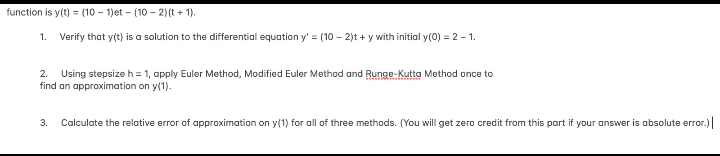# (Solved):   please help asap thank you unction is $$y(t)=\{10-1) e t-(10-2)(t+1)$$ 1. Verify that ...thank you

unction is $$y(t)=\{10-1) e t-(10-2)(t+1)$$ 1. Verify that $$y(t)$$ is a solution to the differential equation $$y^{\prime}=(10-2) t+y$$ with initial $$y(0)=2-1$$. 2. Using stepsize $$\mathrm{h}=1$$, apply Euler Method, Modified Euler Method and Runge-Kutta Method once to find an approximation on $$y(1)$$. 3. Calculate the relative error of approximation on $$y(1)$$ for all of three methods. (You will get zero credit from this part if your answer is absolute error.)

We have an Answer from Expert